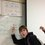# Interesting Limit to Infinity Problem

$\lim_{n\to\infty}\frac{1^{n}+2^{n}+3^{n}+...+n^{n}}{n^{n}}$

alternatively,

$\lim_{n\to\infty}\sum_{i=1}^n{(\frac{i}{n})}^n$

My friends and I have been having a lot of difficulty with this particular problem. We've tried a variety of methods, such as Riemann Integration and L'Hospital's rule, but we've been unsuccessful. After plugging in large values for n we have found an extremely clean answer that we are confident in, but we still have no proof? Any ideas?Note by Logan Dymond
6 years, 2 months ago

MarkdownAppears as
*italics* or _italics_ italics
**bold** or __bold__ bold
- bulleted- list
• bulleted
• list
1. numbered2. list
1. numbered
2. list
Note: you must add a full line of space before and after lists for them to show up correctly
paragraph 1paragraph 2

paragraph 1

paragraph 2

[example link](https://brilliant.org)example link
> This is a quote
This is a quote
    # I indented these lines
# 4 spaces, and now they show
# up as a code block.

print "hello world"
# I indented these lines
# 4 spaces, and now they show
# up as a code block.

print "hello world"
MathAppears as
Remember to wrap math in $ ... $ or $ ... $ to ensure proper formatting.
2 \times 3 $2 \times 3$
2^{34} $2^{34}$
a_{i-1} $a_{i-1}$
\frac{2}{3} $\frac{2}{3}$
\sqrt{2} $\sqrt{2}$
\sum_{i=1}^3 $\sum_{i=1}^3$
\sin \theta $\sin \theta$
\boxed{123} $\boxed{123}$

## Comments

Sort by:

Top Newest

That's a very nice problem indeed! Here's my take.

Fix any positive integer k. Then for all n>k, the sum:

$\frac{1^n + 2^n + \ldots + n^n}{n^n} \ge \frac{(n-k)^n + (n-k+1)^n + \ldots + n^n}{n^n} = \left(1 - \frac k n\right)^n + \left(1 - \frac{k-1}n\right)^n + \ldots + 1.$ (*)

From calculus, we know that for n>k, we have $\left(1 - \frac k n\right)^n \to e^{-k}$ as an increasing sequence in n. [ Proving that it's increasing is a bit tedious, e.g. you can replace n with a continuous variable x and differentiate with respect to x. ] So the RHS tends to $e^{-k} + e^{-(k-1)} + \ldots + 1$ as n tends to infinity. Taking the lim inf of (*) as $n\to\infty$ gives:

$\lim \inf_{n\to \infty} \frac{1^n + 2^n + \ldots + n^n}{n^n} \ge 1 + e^{-1} + e^{-2} + \ldots + e^{-k}.$

Since this holds for each k, the LHS is at least $1 + e^{-1 } +e^{-2} + \ldots = \frac e {e-1}$.

On the other hand, for each n, we have:

$\frac{1^n + 2^n + \ldots + n^n}{n^n} = \left(1 -\frac{n-1}n\right)^n + \left(1 - \frac{n-2}n\right)^n + \ldots + 1 \le e^{-(n-1)} + e^{-(n-2)} + \ldots + 1$

Taking the limsup of both sides gives:

$\lim \sup_{n\to\infty} \frac{1^n + 2^n + \ldots + n^n}{n^n} \le \lim\sup_n (e^{-(n-1)} + e^{-(n-2)} + \ldots + 1) = \frac{e}{e-1}.$

Comparing the lim inf & lim sup of both sides should give us $\frac e {e-1}$.

[ PS. Sorry for using lim inf and lim sup; I had wanted to apply Squeeze theorem directly, but somehow couldn't get it to work. ]

- 6 years, 2 months ago

Log in to reply

Hi sir... How did you come up with the inequality in the first statement?

- 6 years, 2 months ago

Log in to reply

$\lim_{n\to \infty}(\frac{i}{n})^n =\lim_{n\to \infty} (1 -\frac{n-i}{n})^n = e^{-(n-1)}.$

We get:

$\lim_{n\to \infty} \sum_{i=1}^n {(\frac{i}{n})^n} =$ $\lim_{n\to \infty} \sum_{i=1}^n {e^{-(n-i)}} =$ $\lim_{n\to \infty}{ \frac{e-e^{1-n}}{e-1}} = \frac{e}{e-1}.$

---------------------------------------Q.E.D.---------------------------------------------

Is this not correct enough?

- 6 years, 2 months ago

Log in to reply

Your first statement does not make any sense. The answer should be independent of $n$, indicating that your answer is wrong.

You made the mistake of claiming that

$\lim_{n\rightarrow \infty} (1 - \frac{f(n) } { n} ) ^n = e^{- f(n) }$

We know that the statement is true for constants, but not necessarily for functions. For example, if $f(n) = n$, then the expression is always 0, hence the limit is 0, and not $e^{-n}$ whatever that is. It might be true that

$\lim_{n\rightarrow \infty} (1 - \frac{f(n) } { n} ) ^n = \lim_{n \rightarrow \infty} e^{- f(n) },$

but that will require some work too (esp if $\lim f(n)$ does not exist).

Staff - 6 years, 2 months ago

Log in to reply

Thanks, good point!

- 6 years, 2 months ago

Log in to reply

Thematically it's quite similar to the solution provided by C L, but lacks sufficient mathematical rigor. Certainly your work vaguely suggests what the limit could be, as well as a means to prove it, but for the reasons Calvin has explained, it cannot stand as mathematically valid. One needs to be more careful--showing that the limit infimum is bounded below, in my opinion, is the critical step of C L's proof.

- 6 years, 2 months ago

Log in to reply

Thanks, good point!

- 6 years, 2 months ago

Log in to reply

×

Problem Loading...

Note Loading...

Set Loading...